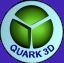INDEX TRIANGLE LIST Tl Variables MATH VIRTUAL GPU CVgpu Variables Downloads Quark 3D graphic library for pocket PC 1/ QUICK OVERVIEW Quark 3D is a compact graphic library for pocket pc. It provide a minimal set of functions to draw 2D and 3D graphics, and is mainly optimized for speed. The main features are: 2D Drawing:- Pixel- Line- Sprite 3D Drawing:- 3D sprite (billboard)- Triangle- Triangle list using flexible vertex and index format. Draw triangles in following modes:- untextured and textured with or without perspective correction- gouraud shading- wireframe- Use Z buffer.- Opaque, alpha, and alpha keyed drawing modes.- Automatic z sort for alpha triangles. For 3D, an immediate drawing mode using separate triangles, or some triangle list using flexible vertex format can be used. Structure ready to support future 3D hardware. Automatic detection of blit function to use for compatibility with standard pocket pc 2003 and previous ppc version ('old' iPaq 36xx to 38xx serie) Provide a mimimal set of math/matrix/vector functions working on integers and highly optimized for speed. Full documentation included in this page, and 15 tutorials. Use a single small dll (size is 52kb) and a single header file. Royalties free for pocket pc applications. 2/ TECHNICAL OVERVIEW Frame Buffer Every graphics are drawn into an offscreen buffer containing z buffer + pixels data. When the scene drawing is completed, a blit function copy this buffer into video memory to display a new frame. Frame buffer size is a linear array on 240*320 32bits unsigned integer value. Each pixel is coded on unsigned integer value as follow: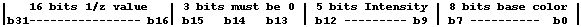b0-b7: 8 bits for base color in fixed palette    - b0-b2 : 3 bits for blue    - b3-b5 : 3 bits for green    - b6-b7 : 2 bits for red b8-b12: 5 bits Intensity for base    - 0 : min light intensity for b0-b7 defined    - 31 : max ligh intensity for b0-b7 defined colorb13-b15: must be 0    Another value will produce bad color for pixelb16-b31: Z buffer value. The Z buffer value contain 1/z (z > 0) scaled by 2^INVTBL_SHIFT (INVTBL_SHIFT = 22) Example: if z = -100 in world 3D coordinate:3D -> 2D projection will convert z to -z -> +100 (using CVGpu::ScrProjection() function)Rasterization will write (2^22 - 1)/100 in b16-b31 = 41943 into z buffer. For this reason, minimum drawable distance is 64 (-CLIPZ_NEAR) that give (2^22 - 1)/64 = 65535, that is the maximum value that can fit on an unsigned 16 bit. Note: near pixels have bigger Z buffer value than far objects. Colors and Textures For every graphics, pixels, lines, sprites, and triangles, Quark use a fixed palette of 256 base colors + 32 intensity shading level for each color. Textures are used to draw triangles or sprites. A texture pixel is stored on 8 bits and allow to code 8 base colors on a fixed palette. For each color there are 32 level of intensity and give at total 256*32 = 8192 color.This is less than pocket pc 2003 display can do, but allow to store textures on 8 bits, and improve performances due to best cache use. Axis +y is up,+x is righ-z is forward in 3D to 2D projection. (3D -> 2D projection 'look' on -z) Axis are oriented direct, (rotating x onto y with trigonometric rotation give +z).This is the same oriention than OpenGL. Object size units Because floats values are avoided for speed considerations, all 3D coordinates are stored on integer values. To obtain a good accuracy in 3D transformations, use objects with 'big' size. For example, 200 or more units length can be used for a car. This depend too of complexity of object. Standard drawing process 1/ - Get object initial vertex position. These positions are defined relatively to object local axis. for example for a sphere, center is at 0,0,0 and vertex are located at radius distance around center. 2/ - Define a transformation matrix. This matrix define how to translate/rotate/scale object to desired location into World 3D coordinates. 3/ - Transform vertex initial positions into new World 3D positions using the matrix and Matrix::TransformVector function. 4/ - The visible part of world is located in view frustrum 3D area. If a bounding box (BBox) is defined for object (3D box including all vertex), test if it cross the view frustrum. In not, the object is not in field of view and there is nothing to display. If it cross, convert all vertex 3D positions to 2D positions using 3D -> 2D projection function (use CVGpu::ScrProjection function). Store the clipping mask m returned by CVGpu::ScrProjection function. This m value define a binary mask depending of the vertex position relative to view frustrum. if m = 0 then vertex is visible into frustrum, else it is not visible and m bits define relative position as followb0 = 1 : hidden at bottomb1 = 1 : hidden at topb2 = 1 : hidden at leftb3 = 1 : hidden at rightb4 = 1 : hidden because too farb5 = 1 : hidden because too near or behind camera note that if b5 = 1, the CVGpu::ScrProjection function do nothing and do not return 2D coordinates, this because is is not possible to transform if z=0 (require a division by 0 or if z to small (1/z become too big and produce overflow), or if z < 0 (negative distance ?) 5/ For each triangle of object to draw, check clipping mask.Skip drawing if all three vertex are hidden at bottom, or top, or left, etc..., else draw. note: if triangle is visible but one of the vertex have b5 = 1 for clipping mask, then it is not possible to draw triangle because the 2D coordinates of the vertex are not available. The solution consist to truncate the triangle with the near clip plane using 3D world coordinates. note: Step 3 to 5 are done by CVGpu::DrawTriangleList function (including near clip truncate).note: this full drawing process is used in tutorial 14 (demo14.cpp) Memory At Init, the CVgpu object allocate about 360k of memory to work (Frame buffer, math table, etc..). If Triangle list or Z sort of faces (alpha) is used, then some additional memory must be allocated. This can be done using CVgpu::SetWorkMemory. 2/ MAIN HEADER Quark 3D use a unique header file for interfacing with applications.This header file contain 2 main objects.The main object is CVGpu, (as 'Virtual graphic processor unit :GPU')This is the object used to draw everything and refresh screen. The second main object is CTriangleList, that manage triangle list containing some vertex + vertex index (triangle) and draw command list. 3/ MAIN HEADER DESCRIPTION The following text describe sequentially every part of quark.h header file.Each functions/variables/defines/enums are explained. Defines and emums Cannot be changed because dll have been compiled with these defined values. // screen size#define SCR_X 240#define SCR_Y 320 Define screen size. // color definition for offscreen bitmap (*VGpu.VScreen)#define GD_BITS 5#define RD_BITS 2#define GR_BITS 3#define BL_BITS 3 GD_BITS define number of bits used to code intensity for a base color. Current value of 5 allow to code 32 values. (2^5). 0 = dark, 31 = max intensity. Note that it is the framebuffer coding. The user must normaly use 0..255 range to define an intensity. RD_BITS = 2 allow to code 4 level of red for each base colorGR_BITS = 3 allow to code 8 level of green for each base colorBL_BITS = 3 allow to code 8 level of blue for each base color // convert RGB 888 to base 8 bits RGB color #define COLOR_RGB (R, G, B) This macro allow to convert a RGB value coded on 3 bytes (RGB888 range [0..255]) to a RGB value coded on 1 byte in RD_BITS GR_BITS BL_BITS format (RGB233). This define a base color. // convert 8 bits intensity value to VScreen format intensity#define COLOR_I ( I) This macro allow to define an Intensity value that can be combined with a base color to obtain a Color+Intensity value. This value can be written directly into framebuffer. example: to obtain yellow color with intensity = 128 (Intensity range is 0..255), use: int Yellow128 = COLOR_RGB(255, 255, 0) | COLOR_I(128) Note that this function is used only to define a color + intensity for PutPixel function. // max clipping values for near and far clipping planes for rasterization #define CLIPZ_NEAR -64#define CLIPZ_FAR -4095 These values define max allowed range of z in world coordinate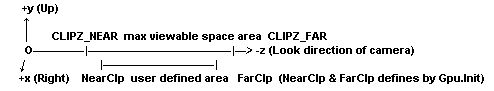notes: - Camera in 3D to 2D projections look on -z, then 3D clipping positions are negatives. - Gpu.Init(int NearClp, int FarClp, ..) function must use NearClp and FarClp in this range with NearClp > FarClp. Passed NearClp and FarClp define z position of clipping planes that must be in [CLIPZ_NEAR..CLIPZ_FAR] range. Triangle that are not in this range will be not displayed. - 3D to 2D projections change sign of Z value to work with positive values in raterization functions. // scale of uv#define UV_MUL 256 Value used to convert floating point texture coordinates to integer coordinates used by system. example: for origin u value of 0.241 -> use 0.241*256 = 61 note: u + k*256 = u. Texture coordinate loop (same than 1.0 value in floating point system). This rule cannot be applyed for a set of texture coordinates if texture is tiled, and the following rule must be applyed. To draw a textured triangle, uv values must be positive without constant offset. A constant offset is a multiple of 256 common to the 3 u or v of each vertex. exemple of u value for triange ABCuA = 515uB = 800uC = 600 this set of values have a constant offset of 512, and must be corrected to :uA = 515 - 512 = 3uB = 800 - 512 = 288uC = 600 - 512 = 88 This allow to reduce uv values and ensure that no overflow will occur in rasterization. It allow too a more accurate rendering of textures. note: The same thing must be done if one of the texture coordinate is negative, then a positive offset of k*256 (k [1..n]) must be added to obtain 3 positives u and v values. This work is done by ConvertUv() function, and internally done by TriangleList::BuildCmdList function. // rasterization modesenum eDrawMode{   DRAW_OPAQUE = 0,  -> opaque drawing   DRAW_KEYED,           -> binary tranparency using transparency color key   DRAW_ALPHA,          -> alpha transparency (50% / 50%)   DRAW_ALPHAKEYED,  -> binary tranparency + alpha transparency mixed}; For DRAW_OPAQUE, the frame buffer is always updated if the z buffer test success. For DRAW_KEYED mode, pixel is drawn and z buffer updated only if texture color != color key. Color key define a texel color that must no be drawn. For DRAW_ALPHA and DRAW_ALPHAKEYED keyed mode, z buffer is tested but not updated. DRAW_ALPHAKEYED mix ALPHA and KEYED mode, then draw an alpha pixel if texture color != color key. This mode is available only for sprites. (ex: ghost on demo2.cpp) note: color key can have a different value for each texture, and must be defined in CTexture.Init function. // drawing mode of trianglesenum eTriangleMode{   TRI_NZC = 0,  -> Z perspective correction disable   TRI_ZC,          -> Z perspective correction enable   TRI_UT,          -> untextured drawing   TRI_WF,         -> wireframe drawing}; Define how to draw triangle. Textured, Textured with perspective correction, untextured, or in wireframe. // error code for functions, stored in ErrorCode if error occuredenum eErrorCode{  ERR_NONE = 0,   -> no error  ERR_MEM = 1, -> function failed to allocate some memory  ERR_TMEM = 2, -> not enough allocated working memory  ERR_UV = 3, -> uv are undefined  ERR_VFMT = 4, -> unknow vertex format  ERR_IFMT = 5, -> unknow index format  ERR_DUPI = 6, -> duplicate intensity value (in vertex + index)  ERR_DUPUV = 7, -> duplicate uv value (in vertex + index)   ERR_F32 = 0x7fffffff, -> force 32 bits enum}; These defines are used by CTriangleList object, and CVGpu DrawTriangleList function. Most of CTriangleList functions return 1 if success, else place one of this error code into CTriangleList::ErrorCode and return 0 if fail. This allow to retrieve why error occured. CVGpu::DrawTriangleList function return directly this error code (return ERR_NONE if success). // texture definition class CTexture{public:  int SizeX;  int SizeY;  unsigned char *Texels;  unsigned int DrawMaskX;  unsigned int DrawMaskY;   int Init(unsigned char *RGB8, int x, int y, int CKey);}; Texture datas are stored on a 8 bit array containing base color of a fixed palette.The init function prepare Texels array and define DrawMaskX and DrawMaskY for rasterization.Texel values are reseted to 0 if initial color = color key. Function define DrawMaskX and DrawMaskY (used for rasterization, must be not modifyed). Return 1 if x and y size are power of 2 and in [16..128] range. x can be not equal to y. If 1 returned, texture can be used to draw some triangles, else it can be used only to draw sprites that do not have size constraints. // rasterization states for drawing typedef struct{  unsigned char DrawMode;  unsigned char DrawColor;  unsigned char DrawIntensity;  unsigned char TestCulling;  unsigned char ZSort;  unsigned char al;  CTexture *Texture;} TRasterState; A rasterization state is a defined hardware state for rendering. Although there is no 3D hardware on pocket pc (not yet), this will allow future compatibility.A state is a set of settings to do on hardware for a given drawing mode. A specific state initialization must be done every time that: - change texture to bind on a triangle- change working mode of z buffer- change drawing mode (opaque, alpha, keyed...)- change culling,- and much more with true 3D hardware.. Setting a new state require to break triangle rendering process and take some time to init. So it is good for speed to batch all triangle using same state, and change states as less as possible.Although there is no 3D hardware on pocket pc, this even allow to increase speed by a better use of memory cache. The CVGpu::DrawTriangleList work like that. When building a triangle list (CTriangleList::BuildCmdList), the function batch all faces that can be drawn with same state, and define a command list. (see DrawTriangleList function) There are 3 default rasterisation states defined in CVgpu object for Text/Lines/Sprites. For triangle drawing, a specific rasterisation state must be defined and assigned to CVgpu.TriangleState pointer. (see BuildCmdList and tutorials for more details) TRasterState parameters: - DrawModeeDrawMode value (opaque, alpha, ..) - DrawColordrawing color (for untextured modes) - DrawIntensitylight intensity, range must be [0..255] - TestCullingIf test culling is enabled (must be by defaut), then triangle that normal vector (perpendicular) is not oriented to camera will be rejected. This is usually the default mode to display a 3D scene, an increase a lot the framerate because it can reject with a simple and fast test about 50% of faces. However, in some case (ex: glass, grid, ..), a face must be visible by the both side (2 sided face). In this case, culling must be disabled. - ZSortThis flag must be set only to draw faces using alpha transparency that overlap. In this case, faces are sorted depending of z distance and not drawn immediatly. When the hole scene using opaque or alphakeyed faces is drawn, then a call to CVgpu::SortDraw function will draw all sorted faces from far to near to ensure correct rendering of alpha. Note: this is significantly more slow than normal drawing, and must be use with a small number of faces.Note: this work only for triangles, and not for sprites. - al (3 bytes)These values are not used, exept to align structure size on a 4 bytes value - TextureDefine ptr to texture to apply in textured mode. // Vertex format used for direct rasterization (DrawTriangle function)typedef struct{  int x, y, z;  int m;  int i;  int u, v;} TVertex; This is the vertex format used for direct triangle drawing, using screen coordinates.x, y, z : must contain screen coordinates (z must be a positive value > -CLIPZ_NEAR) x=0, y=0 -> top left of screenx=SCR_X, y=SCR_Y -> bottom left of screen m : is visibility mask, value is used to define if a set of 3 vertex is visible on screen.i : light intensity of vertex, [0..255] rangeu, v : texture coordinates, must be positive. (see demo5.cpp) Vertex and index format for DrawTriangleList function() // format of vertex in a vertex listenum eVextexFormat{  VF_XYZ = 0,  VF_I = 1,  VF_UV = 2,}; // format of index to define a triangle in a triangle listenum eIndexFormat{  IF_ABC = 0,index format  IF_I = 1,  IF_C = 2,  IF_UV = 4,}; These defines allow to specify a flexible vertex format to draw a triangle list. For example, to draw an object that is not lighted and not textured, only vertex position and vertex index (in a vertex list) are required to draw a face. The vertex format will be:int VertexFormat = VF_XYZ;int IndexFormat = IF_ABC; Then the vertex list will contain only 3 short per vertex, and index list 3 unsigned short per triangle (3 index of vertex in vertex list).In this case, all faces of object will use color and intensity defined in CVGpu->TriangleState. To define a custom color for each face, add a color value in index list and define new index format as follow: int VertexFormat = VF_XYZ;int IndexFormat = IF_ABC | IF_C; To add intensity for each face (face lightening), define int VertexFormat = VF_XYZ;int IndexFormat = IF_ABC | IF_C | IF_I; Or to define intensity for each vertex (vertex lightening), define int VertexFormat = VF_XYZ | VF_I;int IndexFormat = IF_ABC | IF_C; The defined Vertex and index format must be passed to Init function of a CTriangleList object to allocate required memory. Note: The Init function of a CTriangleList object will return error if intensity or uv are defined in both Index and vertex format. class CTriangleList This class used to define a triangle list. short *VertexList;int VertexListSize;int VertexStride; VertexList contain the vertex list (VertexListSize * VertexStride short values).VertexListSize is the size of list in vertex (not in short).VertexStride depend of defined vertex format, and define the size in short used for one vertex. To acces to vertex n, use Data = VertexList[n*VertexStride], or use data access function described below. unsigned short *IndexList;int IndexListSize;int IndexStride; Same coding than vertex list, but contain Index list that define each triangle. IndexList contain the index list (IndexListSize * IndexStride unsigned short values).IndexListSize is the size of list in traingles (not in short).IndexStride depend of defined index format, and define the size in unsigned short for one index. A minimal triangle is defined by 3 index of vertex into Vertex List. The following datas are used for internal work of triangle list There are initialized by Init and BuildCmdList functions. // offset to data into Vertex/Index list, defined by Init function:int VuOfs; -> uv offset in vertex dataint ViOfs; -> intensity offset in vertex dataint IuOfs; -> uv offset in index dataint IiOfs; -> intensity offset in index dataint IcOfs; -> color offset in index data These variables define offset of datas into a verter or index. Value is 0 if data is not available into index/vertex. (depend of defined format) Example: to access to color intensity of vertex n into vertex list, use:int Intensity = VertexList[n*VertexStride + ViOfs]; TRasterState *State; This must contain ptr to a list of rasterization state that will be required to draw the hole triangle list. It can contain only one state if the triangle list use the same rasterization state for all triangles. unsigned short CmdMin;unsigned short *CmdList;int CmdListSize; Command list:A base command use 2 unsigned short: n = unsigned short 0s = unsigned short 1 n : number of triangle to draw using same rasterization states : index in state liste (*CTriangleList::State) of state to use to draw the n triangles defined in triangle list For example, to draw an object with 3 opaque textures, the State list will contain at least 3 rasterization states and CmdList will contain at least 3 commands (6 unsigned short). The CTriangleList::BuildCmdList function batch all faces using same state and define command list. (and alloc required memory for this command).The CmdMin list is a minimal command list defined at CTriangleList::Init. It define a default command that draw all triangle using rasterization state 0 (TRasterState::State) If the BuildCmdList detect that only one state is used, this list is used instead to alloc a new command list with only 2 unsigned short. eErrorCode ErrorCode; Error code is defined with an eErrorCode enum value if one of the CTriangleList function fail and return 0. CTriangleList functions description: int Init (int VFormat, int VCount, int IFormat, int ICount, TRasterState *StateList) - Alloc memory to store vertex and index list, depending of defined vertex and index format.- Define TRasterState::State ptr using passed StateList pointer.- Build a minimal default command list to draw all faces with StateList- Check passed format of vertex/index, return 0 if bad format passed (or vertex/index format not compatible)- return 1 if success int WeldVertex (int WpDelta, int WiDelta, int WuvDelta) Weld vertex is used to remove redundant vertex. If vertex list contain identical vertex, or small variation in position/intensity/uv, then only one vertex is keeped. This allow to increase speed and may remove some visual defaults. The keeped vertex contain averave value of all welded vertex. The maximum variations allowed to weld is define by:WpDelta : variation on positionWiDelta : variation on color intensityWuvDelta : variation on texture uv For example, to weld vertex strictly identical (strictly same position, i, and uv), use:WeldVertex(0, 0, 0); To weld vertex having same position with error max = +/- 1 unit, and strictly same uv and intensity, use:WeldVertex(1, 0, 0); The function fail if it cannot allocate temporary working memory.Warning: The function can change number of vertex in vertex list. The function update vertex index if some vertex are removed, and realloc allocated memory of vertex list to exact required sieze. Note: To test a list of n vertex, the function use a (n^2)/2 loop, and may require lot of time if n is big (use in precalc only, and if the vertex list is 'dirty'). int BuildCmdList (const unsigned short *StateIdList) This function construct a list of command to draw all faces of the triangle list with a minimal count of rasterization states. - Batch all faces using same rasterization state.- Allocate and build list of commands.- Convert uv values to positive values and remove constant offset for a set of uv if uv stored in index list- Remove flat faces. (aligned vertex), and realloc index list to new size if some faces removed. int BuildFNormalList (short *NList, int Stride16) const This function build a list of vector perpendicular to each face. The vector is normalized in size. (size of a normalized vector = TRIGO_MUL = 16384).The NList is filled by vectors (3 short values) using a stride step (in short, not bytes) between each vector. The NList allocated size must be >= CTriangleList::IndexListSize.Function return 0 if cannot allocate temporary working memory. int BuildVNormalList (short *NList, int Stride16) const Same use than BuildFNormalList, but built a vector normal list to each to vertex. Vertex normal is defined using neighbour connected faces. (define medium value vector) The NList allocated size must be >= CTriangleList::VertexListSize.Function return 0 if cannot allocate temporary working memory. void Delete (void); Free memory allocated for triangle list- VertexList- IndexList- CommandList if necessary (not the case if contain only one command and CmdList = CmdMin) Function to access datas into vertex/index list: These functions allow to easily Set/Get datas into Vertex/index list, without taking care of data offset and stride. Write datas functions: void SetABC (int Index, const unsigned short *ABC)  Define some vertex index in IndexListvoid SetXYZ (int Index, const short *XYZ)  Set a vertex position in vertex listvoid SetUV (int Index, const short *UV)  Set 3*uv is uv stored in faces, else 1*uv if stored in vertex.  Note: If uv are stored into vertex, the function SetUV set 2 values (u+v) and the function GetUV get 2 values.void SetI (int Index, int Intensity)  Set a vertex color intensity in vertex or index list.void SetC (int Index, int Color)  Set a color value in index list Read datas functions: void GetABC (int Index, int *ABC) const  Get some vertex index in IndexListvoid GetXYZ (int Index, int *XYZ) const  Get a vertex position in vertex listvoid GetUV (int Index, int *UV) const  Get 3*uv is uv stored in faces, else 1*uv if stored in vertexNote: If uv stored into index, the function SetUV set 6 values (3*(u+v)) and the function GetUV get 6 values too.int GetI(int Index) const  Get a vertex color intensity in vertex or index listint GetC (int Index) const  Get a color value in index list Math functions // angles#define ANGLE_SHIFT 13#define ANGLE2PI (1 << ANGLE_SHIFT)#define DEGREE(a) ((a*46603) >> 11) Definition of system angles for trigonometric functions result.ANGLE_SHIFT and ANGLE2PI define scale to convert angles with: (float) -> (system integer)(2*PI = 6.28...) -> (2*PI = ANGLE2PI = (2^ANGLE_SHIFT) = 8192) The DEGREE macro convert a degree angle value (2*PI = 360 degree) to system angle without using a division. The exact convertion should be DEGREE(a) = (a*ANGLE2PI)/360 // sinus/cosinus result#define TRIGO_SHIFT 14#define TRIGO_MUL (1 << TRIGO_SHIFT) Result scaling for trigonometric functions. Used too to normalize length of vector (including matrix vectors) Then SINUS/COSINUS function return [-TRIGO_MUL..TRIGO_MUL] range instead of [-1.0f..1.0f] range. short SINUS (int a)short COSINUS (int a) Sinus/cosinus functions. Require system angle at input (ANGLE2PI range angle), return a TRIGO_MUL scaled value. Note: Input angle a is masked internally with ANGLE2PI modulo, and any value can be passed. // usefull math functions int Sqrti (int a) Return square root of a. The result is not very accurate (1-2% error max), but function is very fast, and can be used intensively in run time. (for lightening, normalize vector, detect collisions, etc...) int Atan2i (int x, int y) Compute arc tangent. return result in system angle. Relatively fast, but require one division internally. int Log2 (int a) Return log in base 2 of a number. Not fast, use loop. Usually used in precalc mode. // miscelaneous fonctions void ConvertUv (short *UvSet) Adjust a constant offset for a set of 3uv (6 short values) values to get minimal offset value and some positive values. This is based on the fact that u + UV_MUL(=256) = u. (see UV_MUL constant) This function can be used if direct drawing is used. (Trianglelist BuildCmdList call this function automatically). void Normalize (int *N) This function normalize a vector to get norm of vector = TRIGO_MUL. This function is very fast and do not use divisions, however it is not very accurate, but can be use for lightening calculations. void DefUNormal (const int *A, const int *B, const int *C, int *N) This function define a perpendicular vector to plane defined by ABC 3D positions. Result vector lenght is not normalized (length = TRIGO_MUL), however, size is down scaled if length > 32768. (to avoid overflow if try to compute squared length) The vector is oriented for ABC direct. void Itoa (int a, char *aStr) Convert number to string.Same as itoa(), coded because function is not available on windowsCE. // matrix class CMatrix;{  public:  int m;   void LoadIdentity (void); -> init matrix with identity  void Load (const CMatrix *Sm); -> load matrix values  void Mult (const CMatrix *M2); -> multiply matrix M * M2 -> M  void Translate (int x, int y, int z); -> apply translation  void RotateX (int a); -> apply x rotation  void RotateY (int a);  void RotateZ (int a);  void Scale(int s); -> scale 256 = 1.0f, 512 = 2.0f ...  void TransformVector (const int *Src, int *Dest); -> transform vector with rotation + translation  void RotateVector (const int *Src, int *Dest); -> transform vector only with rotation  void InvRotateVector (const int *Src, int *Dest); -> inverse rotation using transposed matrix // get directions of axis into matrix  int *Pos(void) { return &m; }; -> allow to access to position datas  int *Dir(void) { return &m; }; -> dir = z vector  int *Up(void) { return &m; }; -> up = y vector  int *Right(void) { return &m; }; -> left = x vector (if look on -z)}; Classic matrix object. notes: - Rotation vectors in matrix (m[0..8]) are scaled by TRIGO_MUL value.- TransformVector transform a Src vector to Dest vector using matrix transformation.- RotateVector do same thing than TransformVector except that translation is not applyed.- InvRotateVector transform vector with the inverse rotation coded by matrix (used transposed matrix). The translation is ignored. Accessors Pos, Dir, Up, Down allow to access some specific vector into matrix, for position, direction, up and right direction that the matrix is coding. The matrix elements (i,j) into matrix can be accessed directly using mIndex = (i + j*3). 'Virtual' GPU main object class CVGpu // default drawing statesTRasterState TextState -> state used to draw textTRasterState LineState -> state used to draw linesTRasterState SpriteState -> state used to draw sprites Default rasterization states for text/line/sprites. It allow to define color, intensity, texture, etc... to draw text/lines/sprite. These 3 states are initialize with some default value by CVgpu.Init function. TRasterState *TriangleState -> ptr to state used to draw triangle This pointer must point to a user defined rasterization state that will be used to draw triangle. This can be a state define in a triangle list, or another state. If triangle list used, rasterization will use this state to retrieve default value to use if not defined into vertex/index list. Use of a pointer allow to switch very quickly between states by simply changing ptr value. // triangle drawing modeint TriangleMode Define drawing mode of triangle, it must be a eTriangleMode enum value. (see eTriangleMode) // fov/clipping parametersint Zoom This value is used by CVgpu::ScrProjection to transform 3D -> 2D vector. It depend of field of view and is defined by CVgpu::SetFov function. (or CVgpu::Init function that call SetFov internally) It must not be modifyed directly, because clipping plane vectors of frustrum are adjusted depending of this value, or the CVgpu::ClipMsk and CVgpu::CrossFrustrum function will return wrong results. Zoom is defined as follow: with a = (Fov*ANGLE2PI)/(360*2) : a = (field of view)/2 in system units, with Fov in degreeZoom = (COSINUS(ax)*(SCR_X/2))/SINUS(ax) // clipping parametersint ClipNearint ClipFar Local Near and Far clipping plane positions. must be in [CLIPZ_NEAR..CLIPZ_FAR] range. (see CLIPZ_NEAR) When using triangle list drawing, faces are truncated with position of near clip plane, an face having at least one vertex more far that far clip plane are rejected (not truncated) int NFrustrum Contain vector perpendicular (and normalized) to bottom/top/left/right frustrum planes. These vectors are used to define if a vertex is in the view frustrum using directly world 3D coordinates. (using some dot products) These vectors are defines/updated by a call to CVgpu::Init or CVgpu::SetFov functions, and must not be modifyed directly. // transform matrixCMatrix *ViewMatrix Define the matrix that will be used to transform initial coordinates of a vertex to world coordinate. This matrix is used by CVgpu::DrawTriangleList function to transform vertex of triangle list. // frame counterint FrameCounter This value count number of displayed frames since program start. // frame bufferunsigned int *FrameBuffer Contain address of offscreen buffer for the hole screen. (see Frame Buffer description) // initsint Init (int NearClp, int FarClp, int Fov) Init CVgpu object. Alloc memory for frame buffer, math precalc tables, etc (about 360k required). If define too near clip/far clip plane position and field of view. void Delete (void) Free memory allocated by CVgpu::Init + CVgpu::SetWorkMemory functions // memory initint SetWorkMemory (int MaxVertex, int MaxFSort) This function allow to reserve once some memory for internal work of DrawTriangleList function and Z sort of faces (use hach table) The MaxVertex parameter must define the size of the biggest list of vertex that will be used in a triangle list when CVgpu::DrawTriangleList function will be called. It not enough memory is reserved, DrawTriangleList function will fail and return ERR_TMEM error code. The MaxFSort parameter must define the maximal count of sorted triangle that could be displayed on a frame. Usually, only faces using alpha transparency must be Z sorted for a correct rendering. (may be not necessary in all cases). For example:To display a scene using 20 objects using each 10 alpha faces that require Z sort:If only 5 object can be visible at a time on a frame, maximum face to sort will be 5*10 = 50.MaxFSort must be >= 50 to sort all the faces. In practize, a big value can be set (ex 1000), even if it is too lot. If value is too low, face that cannot be sorted will be not displayed. // text drawingvoid PrintXY (char *Str, int x, int y) Print a string at xy position. (ascii)Color and Intensity can be defined in CVgpu::TextState. void PrintFps (unsigned int Ticks) Print framerate at top left of screen.The current time in Tick (milliseconds) must be passed. // pixel drawingvoid PutPixel (int x, int y, int Color) Draw a pixel at xy position.Z buffer value is ignored.Color must define base pixel color + intensity (concatainated value), COLOR_I macro can be used. note: this is the only function that work directly with color + intensity (No TRasterState defined for pixels) // line drawingvoid DrawLine (int *A, int *B) - Draw a line from A to B, A and B specify xyz screen positions.- Z buffer is tested/updated. z value of a and b must be positive > -CLIPZ_NEAR- Color and Intensity can be defined in CVgpu::LineState. // sprite drawing void DrawSprite (int x, int y)- Draw a sprite at xy screen position, using native texture size.- Texture/intensity must be defined in CVgpu::SpriteState.- Z buffer value is ignored. void DrawSpriteScale (int x, int y, int Scale)  Same than DrawSprite except than a scaling ratio can be defined.  Scaling ratio must be scaled by 256. Ex = 256 -> *1.0f, 512 -> *2.0f, 128 -> *0.5f void DrawSpriteScaleZb (const int *ScrTl, const int *ScrBr, int z)  This sprite mode allow to mix sprites with 3D objects (ex: billboard)  ScrTl must specify screen top left position (can be 2d vector, ScrTl not read)  ScrBr must specify screen bottom right position (can be 2d vector, ScrBr not read).  z must specify z buffer value (positive > -CLIPZ_NEAR) The sprite is scaled to fit in defined top/left Bottom/right positions. Z buffer is updated. Texture/intensity must be defined in CVgpu::SpriteState. // direct triangle drawing. use screen coordinatesvoid DrawTriangle (TVertex *A, TVertex *B, TVertex *C) Draw a triangle using screen coordinate and valid z buffer values (positive > -CLIPZ_NEAR) TVertex.m must contain a valid clipping mask. The clipping mask is returned by CVgpu::ScrProjection function. It can be defined too using CVgpu::ClipMsk function. note: forcing to 0 the clipping mask of ABC will force drawing of face. But this will decrease speed because of execution of some extra code for faces that are out of screen. // triangle list. use 3D world coordinates and ViewMatrixeErrorCode DrawTriangleList (const CTriangleList *Tl) Draw a triangle list (CTriangleList object). Note: Face crossing near clip plane are automatically truncated.Note: This function require some previously allocated memory to work (see CVgpu::SetWorkMemory) Return ERR_NONE if success, else other error code. // Z Sort of triangles for alpha transparency modes void SortReset (void)Clear the Z sort hach table (do not free memory allocated by CVgpu::SetWorkMemory). SortReset must be called once at init to clear hach table. If the hach table is full, new faces requiring Z sort will be ignored and not drawn. void SortDraw (void)Draw faces sorted in hach table, and clear hach table (for next frame). This function must be called on time at scene draw end, when all unsorted faces (opaque faces) have been drawn. // frame buffervoid FlushFB (unsigned int VAddr, int ClearColor) Copy virtual screen into video memory, clear z buffer (to 0) and VScreen pixels with ClearColor. // 3D to 2D transform int SetFov (int Fov) - Define field of view (Fov in degree)- Define projection Zoom value- Define normal to clipping planes of view frustrum (CVgpu::NFrustrum)- Valid Fov range is 8 - 120 (in degree)- If success, return 1/2 of Fov in system angle units, else 0 int ScrProjection (const int *Pos3D, int *ScrPos) - Transform 3D vertex position into 2D screen position.- Return clipping mask (see step 4 of drawing process) Note: if Pos3D z value > CLIPZ_NEAR, then the function set bit b5 (32) of clipping mask and do not transform vector. (explained in step 4 of drawing process) // frustrum clippingint SetNFClip (int NearClp, int FarClp) Define position of near and far clipping planes.NearClp and FarClp must be in [CLIPZ_NEAR..CLIPZ_FAR] range, return 1 if success // clipping testint ClipMsk (const int *wPos) This function can be used to define a vertex position relativly to frustrum. (clipping mask) Note: For triangle vertex, it is better to use result returned by CVgpu::ScrProjection function, because a more fast algorithm is used if vertex is in frustrum (just compare with screen limits). But ClipMsk() can be used to know if an object that we don't need to draw is in frustrum (ex:Bounding box). In this case, use of CVgpu::ClipMsk function if more fast than using CVgpu::ScrProjection int CrossFrustrum (const int *Box) Return 1 if bounding box of an object cross view frustrum (see demo14.cpp for use). A bounding box is a virtual box big enough to include in space all vertex of an object.If the bounding box do not cross view frustrum, then we can be sure that the object is not visible an so can skip drawing process for this object. This is a speed optimization technique. The bounding box must contain 8 vectors, and have minimal 3D size to contain all vertex of an 3D object. // internal work int ZcErrorMax Drawing with corrected perspective is more slow than without. But sometime distorsion is very low (Low Error for small or far faces). In this case, to increase speed it is better to disable correction. ZcErrorMax define the max allowed error on perspective correction. If Error < ZcErrorMax then perspective correction is disabled. Default value is 0: correction is always applyed, even if not required. It can be modifyed to increase speed. A good range is 200 to 1500 200: low error allowed1500: big error allowed An example of use is described in demo14.cpp Downloads Pocket PC Quark library is free for pocket pc, it can be downloaded at  www.pocketgear.com/software_detail.asp?id=12370 It contain tutorials sources, library and dll, for pocket pc and pc. Project files for Visual c++ and embedded visual c++ are included. The compiled examples (contain .exe only) for pocket pc can be download here. The .zip content must be unzipped in a /Quark3D/ directory on the root of your pocket pc (It will not work in another directory, due to use of absolute path on ppc). Then test Demo0 to Demo14 using the ppc file explorer. Click on screen to exit each demo. The compiled examples for PC can be downloaded here. Unzip the content in a directory and select a demo. Note that on the pc, the framerate is timed to 25fps.   HP Jornada 680/690 Quark library is free for hp Jornada 680/690, it can be downloaded at  www.pocketgear.com/software_detail.asp?id=12413 It contain tutorials sources, library and dll, for hpc and pc. Project files for Visual c++ and embedded visual c++ are included. The compiled examples (contain .exe only) for Jornada 680/690 can be download here. The .zip content must be unzipped in a /Quark3D/ directory on the root of your jornada (It will not work in another directory, due to use of absolute path on ppc). Then test Demo0 to Demo14 using the jornada file explorer. Click on screen or press escape to exit each demo. The compiled examples of jornada demos for PC can be downloaded here. Unzip the content in a directory and select a demo. Note that on the pc, the framerate is timed to 25fps. Notes about Jornada 680/690 version: Some of these demos have been targeted to run on pocket pc having arm cpu. (200-400 mhz cpu)Jornada 680/690 use a slower sh3 cpu, at only 133mhz. And access to video memory on this machine is very slow, even with using direct access to video memory (about 3mb/second max). Then, demo 10, 12, 13, 14 are slow. Demo14 is a benchmark and is very slow, and perform an average of 3 fps and bench score of 61. However, some game using low count of triangles and simple geometry should be possible on jornada with a reasonable framerate between 10 to 15 fps. AVAILABLE TUTORIALS: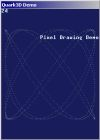Pixel drawing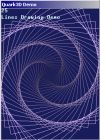Lines drawing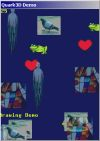2D Sprites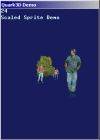2D Scaled sprites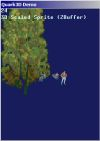3D sprites (billboard)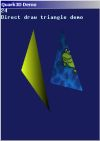Simple triangle drawing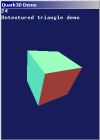Cube drawing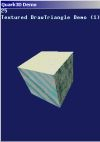Textured cube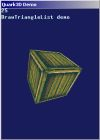Textured cube using triangle list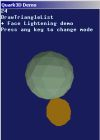Face lightening tutorial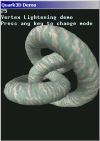Vertex lightening tutorial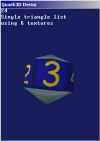Triangle list using multi textures.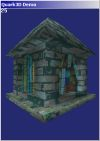Alpha transparency and face sort tutorial.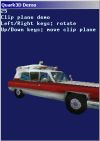Clipping plane demo.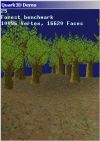High polygon scene. Benchmark your pocket PC.